# RD Sharma Solutions for Class 9 Maths Chapter 18 Surface Area and Volume of Cuboid and Cube Exercise 18.1

Class 9 Chapter 18 – Surface Area and Volume of Cuboid and Cube Exercise 18.1 solutions are provided only here. In this exercise, students will learn about Surface Area of a Cuboid and Cube. Students must have a strong conceptual knowledge in this subject which will be beneficial for them in their future studies. RD Sharma solutions for Class 9 are created by expert subject teachers in an interactive and engaging manner so that students can build interest in Maths. Students must work hard and practice RD Sharma Solution for Class 9 on a regular basis to prepare more efficiently.

## Download PDF of RD Sharma Solutions for Class 9 Maths Chapter 18 Surface Area and Volume of Cuboid and Cube Exercise 18.1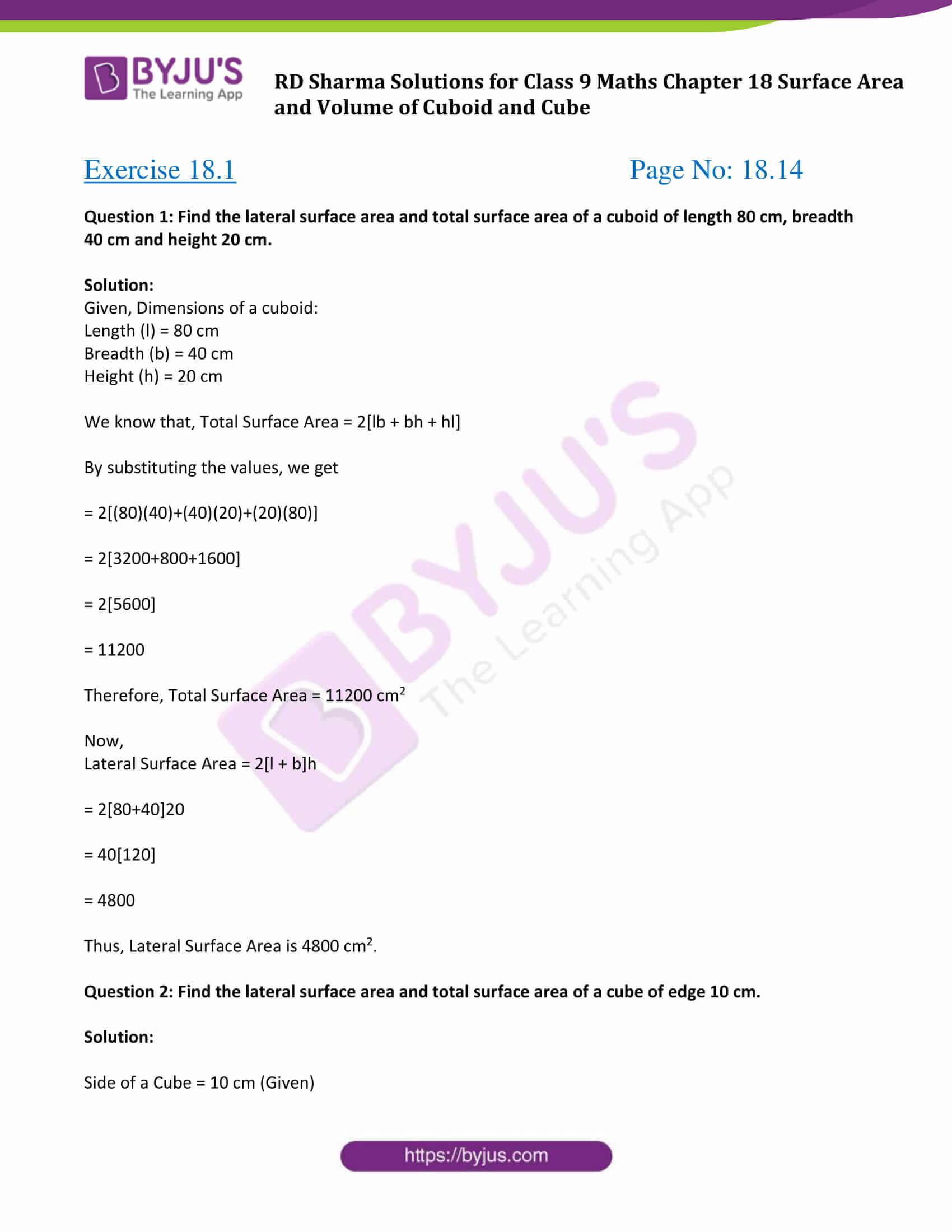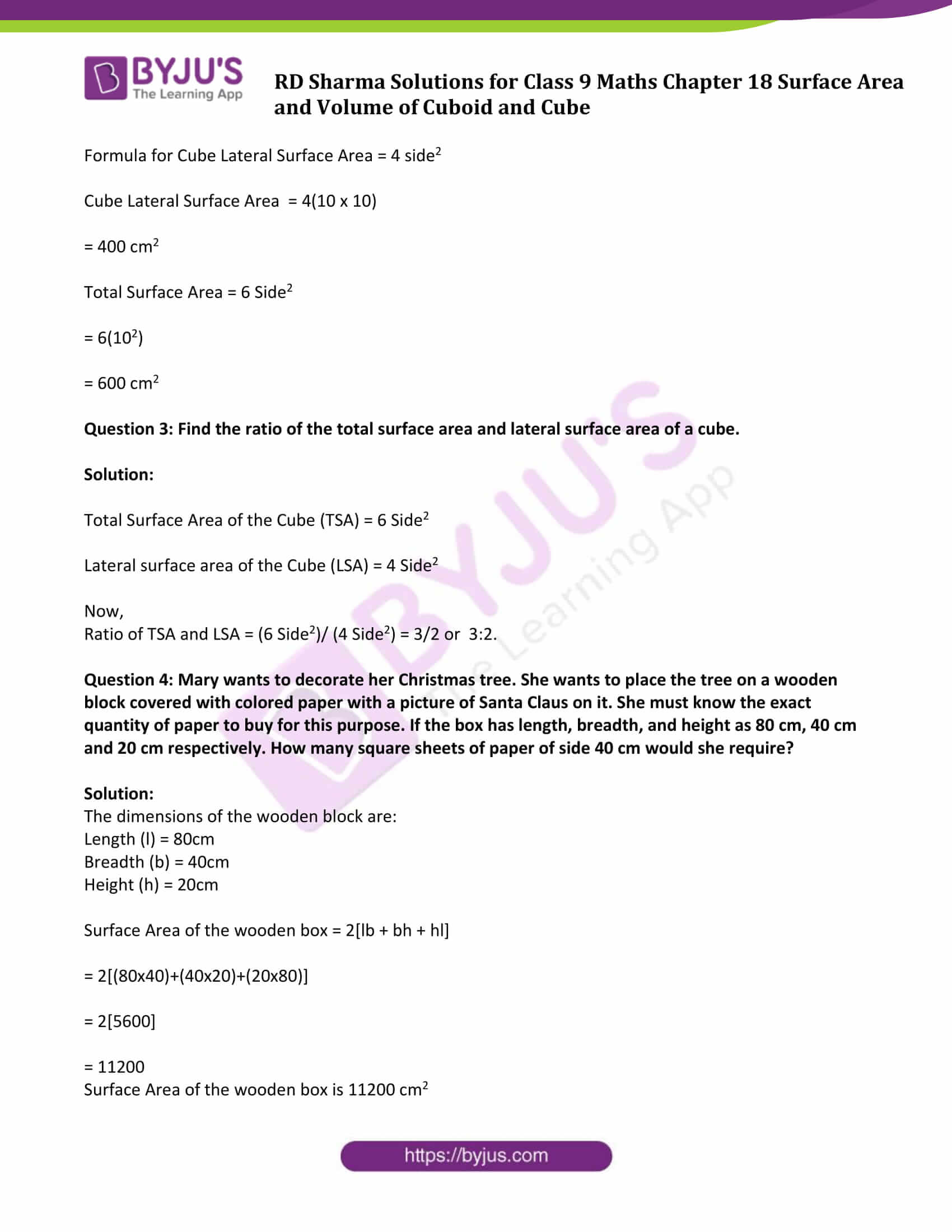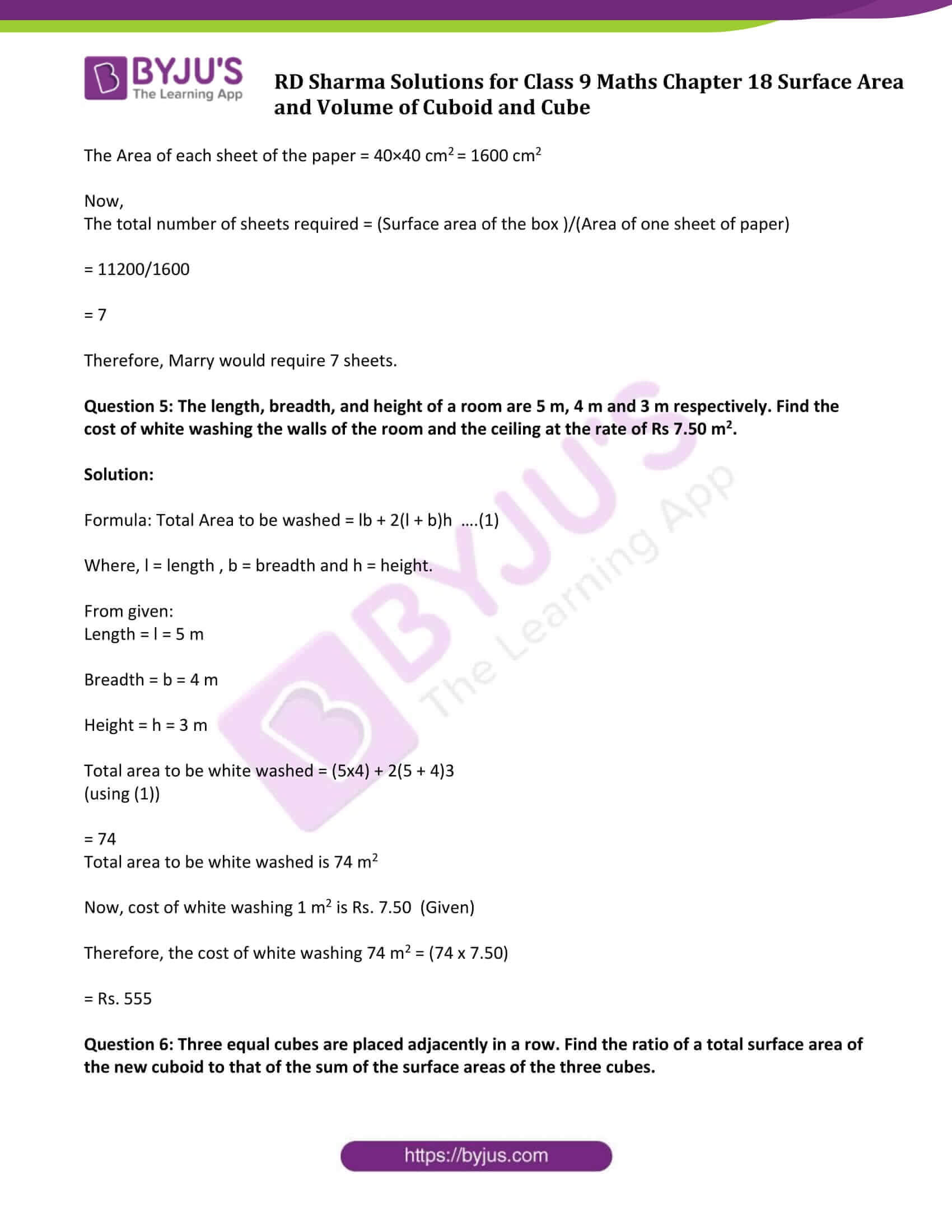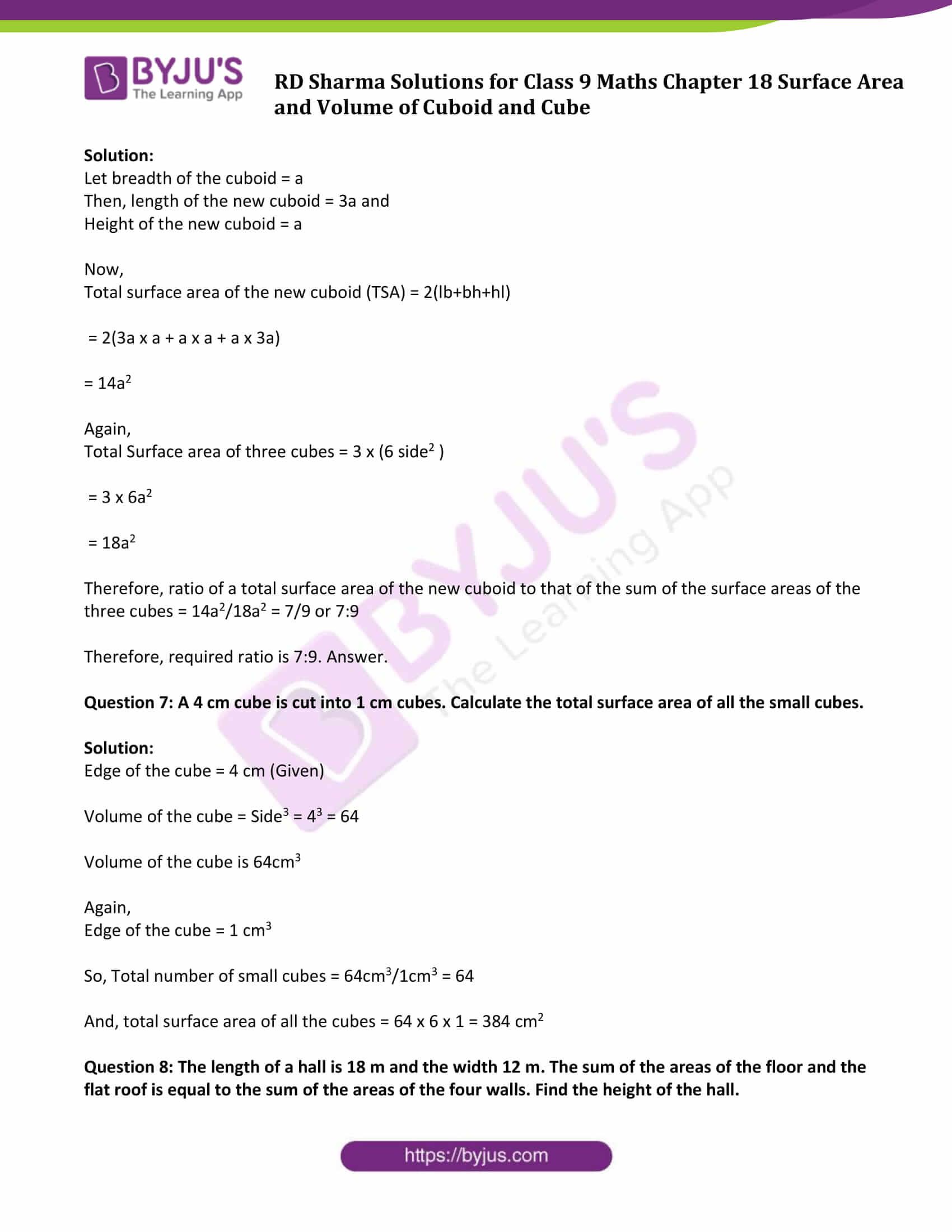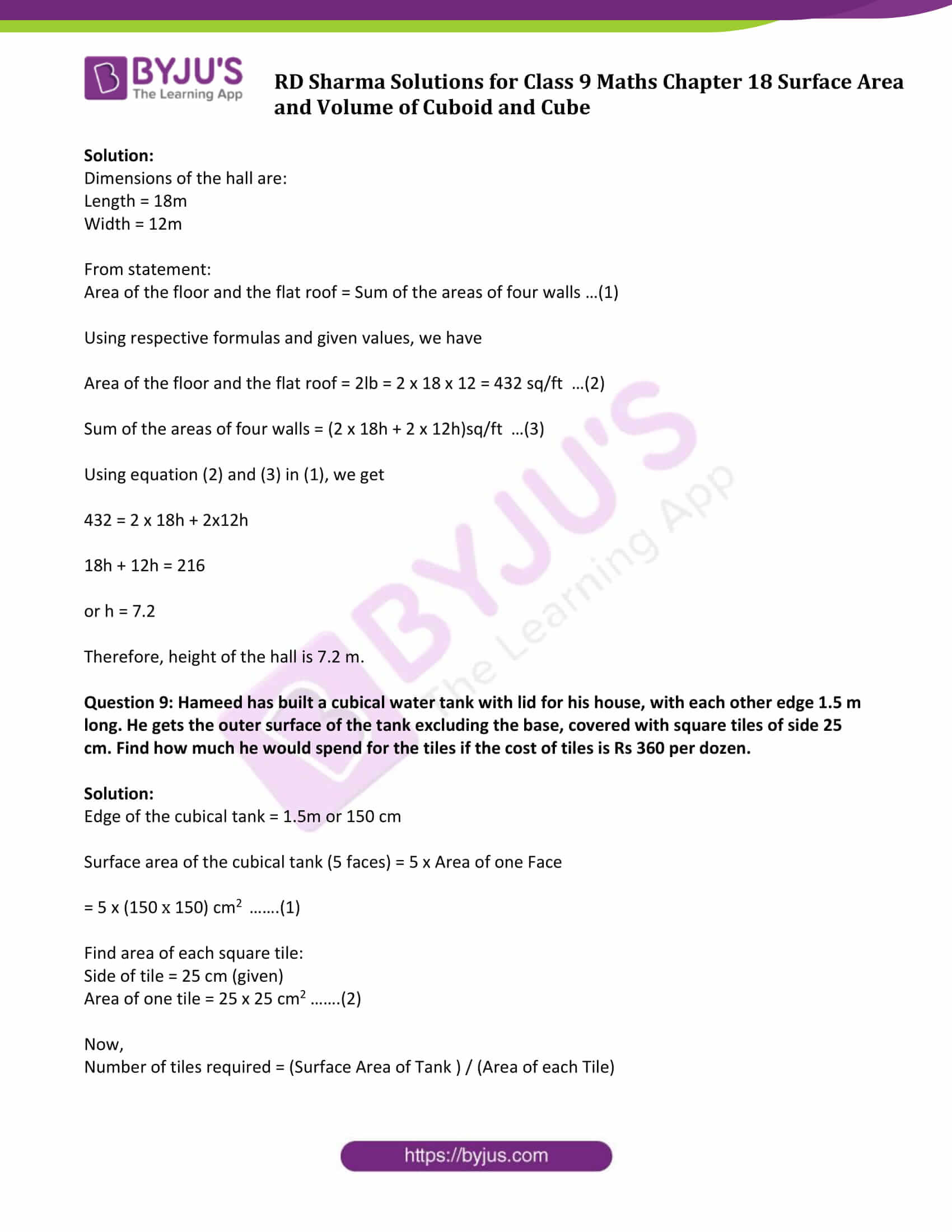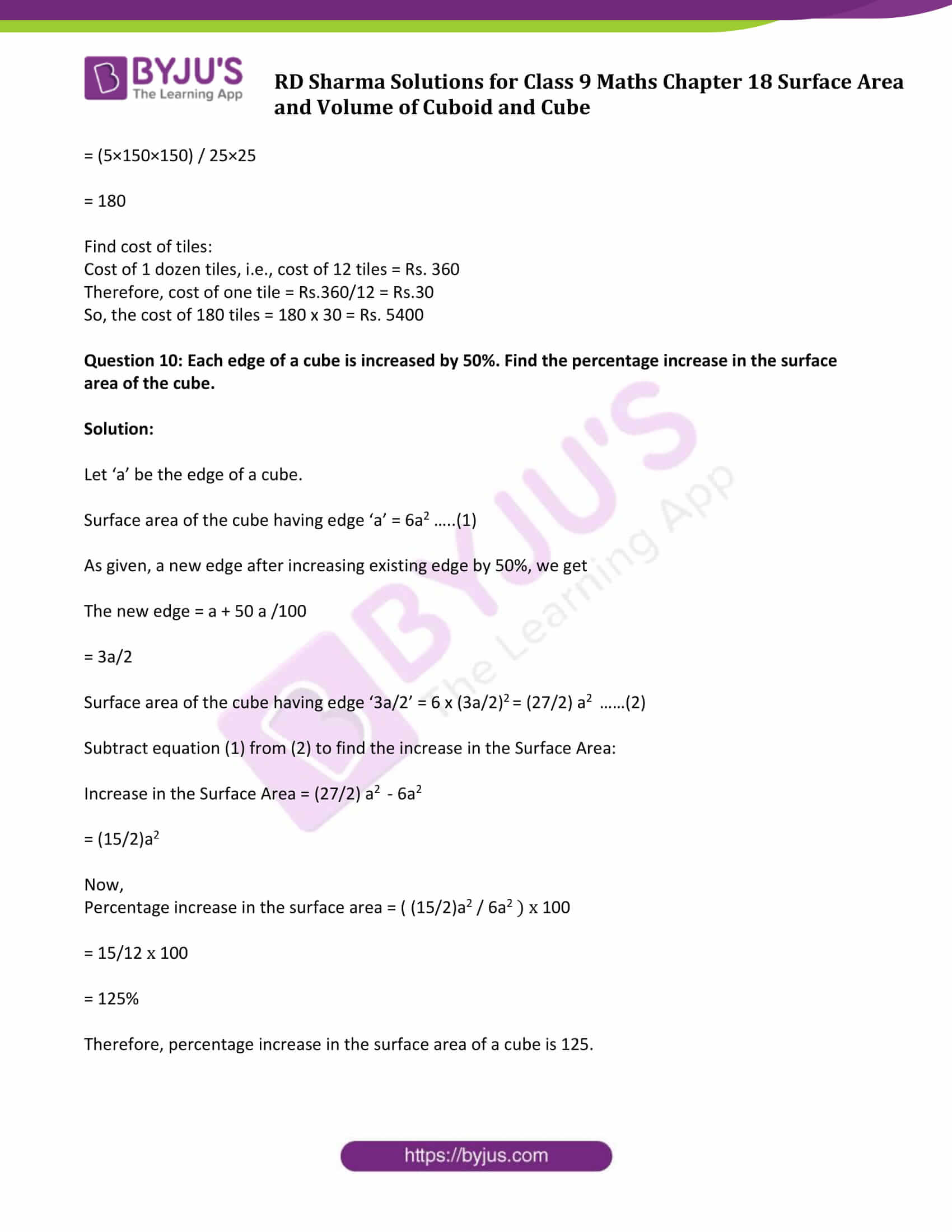### Access Answers to Maths RD Sharma Solutions for Class 9 Chapter 18 Surface Area and Volume of Cuboid and Cube Exercise 18.1 Page number 18.14

Question 1: Find the lateral surface area and total surface area of a cuboid of length 80 cm, breadth 40 cm and height 20 cm.

Solution:

Given, Dimensions of a cuboid:

Length (l) = 80 cm

Height (h) = 20 cm

We know that, Total Surface Area = 2[lb + bh + hl]

By substituting the values, we get

= 2[(80)(40)+(40)(20)+(20)(80)]

= 2[3200+800+1600]

= 2

= 11200

Therefore, Total Surface Area = 11200 cm2

Now,

Lateral Surface Area = 2[l + b]h

= 2[80+40]20

= 40

= 4800

Thus, Lateral Surface Area is 4800 cm2.

Question 2: Find the lateral surface area and total surface area of a cube of edge 10 cm.

Solution:

Side of a Cube = 10 cm (Given)

Formula for Cube Lateral Surface Area = 4 side2

Cube Lateral Surface Area = 4(10 x 10)

= 400 cm2

Total Surface Area = 6 Side2

= 6(102)

= 600 cm2

Question 3: Find the ratio of the total surface area and lateral surface area of a cube.

Solution:

Total Surface Area of the Cube (TSA) = 6 Side2

Lateral surface area of the Cube (LSA) = 4 Side2

Now,

Ratio of TSA and LSA = (6 Side2)/ (4 Side2) = 3/2 or 3:2.

Question 4: Mary wants to decorate her Christmas tree. She wants to place the tree on a wooden block covered with colored paper with a picture of Santa Claus on it. She must know the exact quantity of paper to buy for this purpose. If the box has length, breadth, and height as 80 cm, 40 cm and 20 cm respectively. How many square sheets of paper of side 40 cm would she require?

Solution:

The dimensions of the wooden block are:

Length (l) = 80cm

Height (h) = 20cm

Surface Area of the wooden box = 2[lb + bh + hl]

= 2[(80×40)+(40×20)+(20×80)]

= 2

= 11200

Surface Area of the wooden box is 11200 cm2

The Area of each sheet of the paper = 40×40 cm2 = 1600 cm2

Now,

The total number of sheets required = (Surface area of the box )/(Area of one sheet of paper)

= 11200/1600

= 7

Therefore, Marry would require 7 sheets.

Question 5: The length, breadth, and height of a room are 5 m, 4 m and 3 m respectively. Find the cost of white washing the walls of the room and the ceiling at the rate of Rs 7.50 m2.

Solution:

Formula: Total Area to be washed = lb + 2(l + b)h ….(1)

Where, l = length , b = breadth and h = height.

From given:

Length = l = 5 m

Breadth = b = 4 m

Height = h = 3 m

Total area to be white washed = (5×4) + 2(5 + 4)3

(using (1))

= 74

Total area to be white washed is 74 m2

Now, cost of white washing 1 m2 is Rs. 7.50 (Given)

Therefore, the cost of white washing 74 m2 = (74 x 7.50)

= Rs. 555

Question 6: Three equal cubes are placed adjacently in a row. Find the ratio of a total surface area of the new cuboid to that of the sum of the surface areas of the three cubes.

Solution:

Let breadth of the cuboid = a

Then, length of the new cuboid = 3a and

Height of the new cuboid = a

Now,

Total surface area of the new cuboid (TSA) = 2(lb+bh+hl)

= 2(3a x a + a x a + a x 3a)

= 14a2

Again,

Total Surface area of three cubes = 3 x (6 side2 )

= 3 x 6a2

= 18a2

Therefore, ratio of a total surface area of the new cuboid to that of the sum of the surface areas of the three cubes = 14a2/18a2 = 7/9 or 7:9

Therefore, required ratio is 7:9. Answer.

Question 7: A 4 cm cube is cut into 1 cm cubes. Calculate the total surface area of all the small cubes.

Solution:

Edge of the cube = 4 cm (Given)

Volume of the cube = Side3 = 43 = 64

Volume of the cube is 64cm3

Again,

Edge of the cube = 1 cm3

So, Total number of small cubes = 64cm3/1cm3 = 64

And, total surface area of all the cubes = 64 x 6 x 1 = 384 cm2

Question 8: The length of a hall is 18 m and the width 12 m. The sum of the areas of the floor and the flat roof is equal to the sum of the areas of the four walls. Find the height of the hall.

Solution:

Dimensions of the hall are:

Length = 18m

Width = 12m

From statement:

Area of the floor and the flat roof = Sum of the areas of four walls …(1)

Using respective formulas and given values, we have

Area of the floor and the flat roof = 2lb = 2 x 18 x 12 = 432 sq/ft …(2)

Sum of the areas of four walls = (2 x 18h + 2 x 12h)sq/ft …(3)

Using equation (2) and (3) in (1), we get

432 = 2 x 18h + 2x12h

18h + 12h = 216

or h = 7.2

Therefore, height of the hall is 7.2 m.

Question 9: Hameed has built a cubical water tank with lid for his house, with each other edge 1.5 m long. He gets the outer surface of the tank excluding the base, covered with square tiles of side 25 cm. Find how much he would spend for the tiles if the cost of tiles is Rs 360 per dozen.

Solution:

Edge of the cubical tank = 1.5m or 150 cm

Surface area of the cubical tank (5 faces) = 5 x Area of one Face

= 5 x (150 x 150) cm2 …….(1)

Find area of each square tile:

Side of tile = 25 cm (given)

Area of one tile = 25 x 25 cm2 …….(2)

Now,

Number of tiles required = (Surface Area of Tank ) / (Area of each Tile)

= (5×150×150) / 25×25

= 180

Find cost of tiles:

Cost of 1 dozen tiles, i.e., cost of 12 tiles = Rs. 360

Therefore, cost of one tile = Rs.360/12 = Rs.30

So, the cost of 180 tiles = 180 x 30 = Rs. 5400

Question 10: Each edge of a cube is increased by 50%. Find the percentage increase in the surface area of the cube.

Solution:

Let ‘a’ be the edge of a cube.

Surface area of the cube having edge ‘a’ = 6a2 …..(1)

As given, a new edge after increasing existing edge by 50%, we get

The new edge = a + 50 a /100

= 3a/2

Surface area of the cube having edge ‘3a/2’ = 6 x (3a/2)2 = (27/2) a2 ……(2)

Subtract equation (1) from (2) to find the increase in the Surface Area:

Increase in the Surface Area = (27/2) a2 – 6a2

= (15/2)a2

Now,

Percentage increase in the surface area = ( (15/2)a2 / 6a2 ) x 100

= 15/12 x 100

= 125%

Therefore, percentage increase in the surface area of a cube is 125.

## RD Sharma Solutions for Class 9 Maths Chapter 18 Surface Area and Volume of Cuboid and Cube Exercise 18.1

Class 9 Maths Chapter 18 Surface Area and Volume of Cuboid and Cube Exercise 18.1 is based on the following topics:

• Surface Area of a Cuboid
• Surface Area of a Cube
• Lateral Surface Area of a Cuboid
• Lateral Surface Area of a Cube